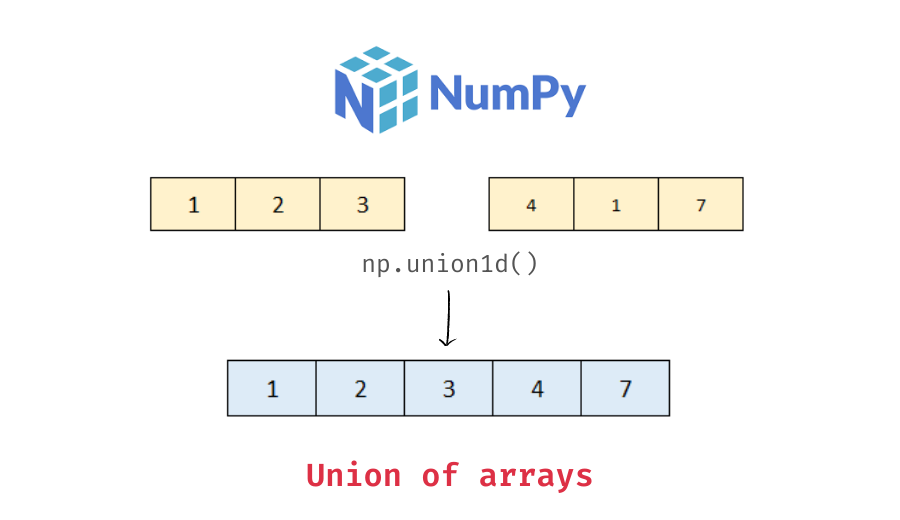# Python – Get union of two numpy arrays

In this tutorial, we will look at how to get the union of two numpy arrays with the help of some examples.

You can use the numpy `union1d()` function to find the union of two arrays. Pass the two arrays as arguments to the function. The following is the syntax:

```import numpy as np
union_ar = np.union1d(ar1, ar2)```

It returns the unique, sorted array of values that are in either of the two input arrays. Note that if the input arrays are not 1d then they are flattened before applying the union operation.

Let’s look at some examples of using the numpy union1d() function.

Pass the two arrays as arguments to the numpy union1d() function.

```import numpy as np

# create two 1d arrays
ar1 = np.array([1, 3, 3, -2, 0, 0, 1])
ar2 = np.array([4, 1, 2, 7])
# union of ar1 and ar2
ar_union = np.union1d(ar1, ar2)
# display the union array
print(ar_union)
# display the type of ar_union
print(type(ar_union))```

Output:

```[-2  0  1  2  3  4  7]
<class 'numpy.ndarray'>```

Here, we created two 1d numpy arrays and then applied the union1d() function. Note that the returned array contains the unique values present in both the arrays in a sorted manner.

If the arrays are not 1d, they are flattened first, and then the union operation is applied. For example, if you apply the union1d() function on two 2d arrays:

📚 Data Science Programs By Skill Level

Introductory

Intermediate ⭐⭐⭐

🔎 Find Data Science Programs 👨‍💻 111,889 already enrolled

Disclaimer: Data Science Parichay is reader supported. When you purchase a course through a link on this site, we may earn a small commission at no additional cost to you. Earned commissions help support this website and its team of writers.

```# create two 2d arrays
ar1 = np.array([[1, 3],
[3, 2]])
ar2 = np.array([[2, 3],
[0, 5]])
# union of ar1 and ar2
ar_union = np.union1d(ar1, ar2)
# display the union array
print(ar_union)```

Output:

`[0 1 2 3 5]`

You can see that we get a 1d numpy array containing the unique values in both the arrays as output.

You can only get the union of two numpy arrays at a time with the np.union1d() function.

To get the union of more than two arrays, you can use the `np.union1d()` function with the `functools` `reduce()` function. See the example below:

```from functools import reduce

# create three 1d numpy arrays
ar1 = np.array([1, 2, 2])
ar2 = np.array([2, 3])
ar3 = np.array([5, 1, 7])
# union of three arrays
ar_union = reduce(np.union1d, (ar1, ar2, ar3))
# display the union array
print(ar_union)```

Output:

`[1 2 3 5 7]`

We get the unique values from the three arrays as a numpy array.

For more on the numpy union1d() function, refer to its documentation.

With this, we come to the end of this tutorial. The code examples and results presented in this tutorial have been implemented in a Jupyter Notebook with a python (version 3.8.3) kernel having numpy version 1.18.5

•